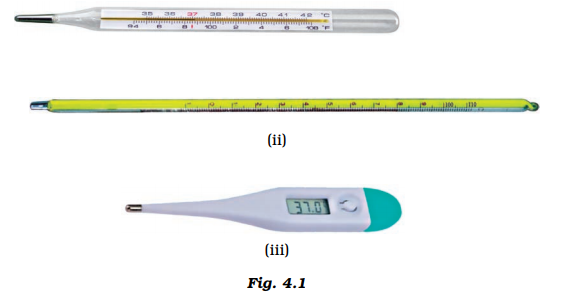# Boojho has three thermometers as shown in Figure. He wants to measure the temperature of his body and that of boiling water. Which thermometer (s) should he choose? (a) Thermometer (i) or (iii) for measuring body temperature and (ii) for measuring the temperature of boiling water. (b) Thermometer (i) for measuring the temperature of both. (c) Thermometer (ii) for measuring the temperature of both. (d) Thermometer (iii) for measuring the temperature of both.Answer: (a) Thermometer (i) or (iii) for measuring body temperature and (ii) for measuring the temperature of boiling water.

Image (i) and (iii) are of clinical thermometers use to measure body temperature and image (ii) is of laboratory thermometers which is used to measure room temperature.

## Thermometer

A thermometer is a device used for measuring temperature. This ice-covered thermometer shows that the temperature is about 0 degrees Celsius, or 32 degrees Fahrenheit.

• Thermometers work by utilizing the physical properties of various substances.
• For example, liquids and solids expand as they heat up and contract when they cool down. Similarly, gases increase in pressure as they heat up and decrease as they cool down.
• A thermometer contains two essential elements: a sensor that detects a change in temperature and a device that communicates that temperature to the person reading it.(0)(0)